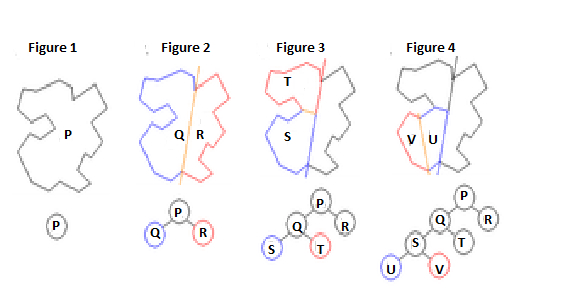# BSP Trees in Data Structure

Data Structure AlgorithmsAnalysis of AlgorithmsAlgorithms

In computer science, a method known as binary space partitioning (BSP) is implemented for recursively subdividing a space into two convex sets by implementing hyperplanes as partitions. This process of subdividing provides rise to a representation of objects within the region in the form of a tree data structure known as a BSP tree.

Binary space partitioning was invented in the context of 3D computer graphics in 1969, where the structure of a BSP tree permits for spatial information about the objects in a scene that is useful in rendering, such as objects being ordered from front-to-back with respect to a viewer at a given location, to be accessed quickly. Other applications of BSPinclude:accomplishing geometrical operations with shapes (constructive solid geometry) in CAD, collision detection in 3D video games and robotics, ray tracing, and other applications that involve the handling of complex spatial scenes.

## Overview

Binary space partitioning is treated as a generic process of recursively dividing a scene into two until the partitioning satisfies one or more requirements. It can be viewed as a generalisation of other spatial tree structures such as k-d trees and quadtrees, one where hyperplanes that partition the space may have any orientation, instead of being aligned with the coordinate axes as they are in k-d trees or quadtrees. When implemented in computer graphics to render scenes composed of planar polygons, the partitioning planes are frequently selected to coincide with the planes defined by polygons in the scene. The specific choice of partitioning plane and criterion for completing the partitioning process varies depending on the purpose of the BSP tree. For example, in computer graphics rendering, the scene is divided until and unless each node of the BSP tree consists of only polygons that can render in arbitrary order. When back-face culling is implemented, each node therefore consists of a convex set of polygons, whereas when rendering double-sided polygons, each node of the BSP tree consists of only polygons in a single plane. In collision detection or ray tracing, a scene can be divided up into some primitives on which collision or ray intersection tests are straightforward.

## GenerationThe canonical implementation of a BSP tree is for rendering polygons (that are double-sided, that is, without back-face culling) with the help of the painter's algorithm. Each polygon is designated with a front side and a back side which could be chosen arbitrarily and only affects the structure of the tree but not the required result. Such a tree is built from an unsorted list of all the polygons in a scene. The recursive algorithm for building a BSP tree from that list of polygons is

• Select a polygon A, from the list.
• Build a node N in the BSP tree, and add A to the list of polygons at that node.
• For list's each other polygon
• If that polygon is located wholly in front of the plane containing A, move that polygon to the list of nodes in front of A.
• If that polygon is located wholly behind the plane containing A, move that polygon to the list of nodes behind P.
• If that polygon is intersected by the plane consisting of A, split it into two polygons and move them to the respective lists of polygons located behind and in front of P.
• If that polygon is located within the plane containing A, add it to the list of polygons at node N.
• Apply this algorithm to the list of polygons located in front of A.
• Apply this algorithm to the list of polygons located behind A.